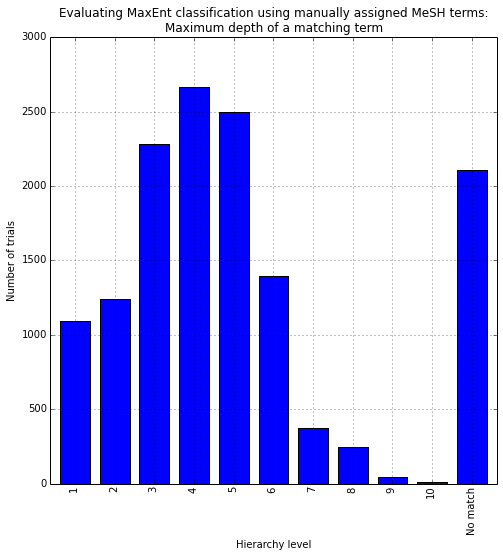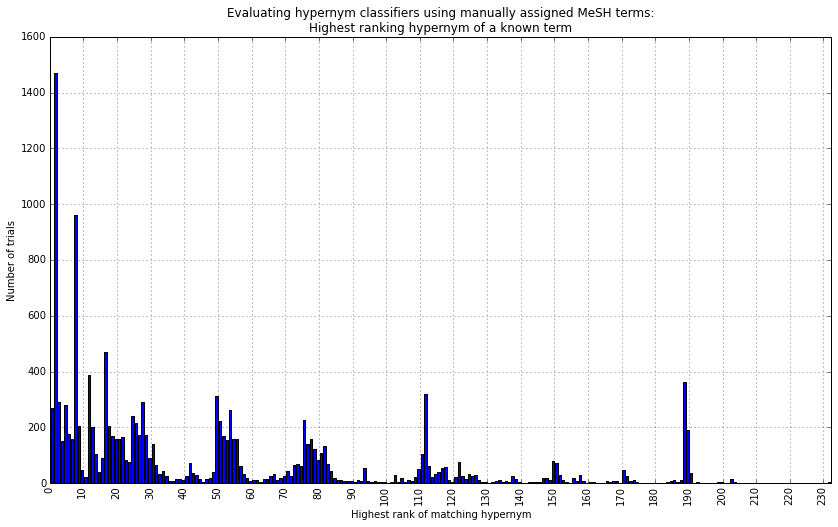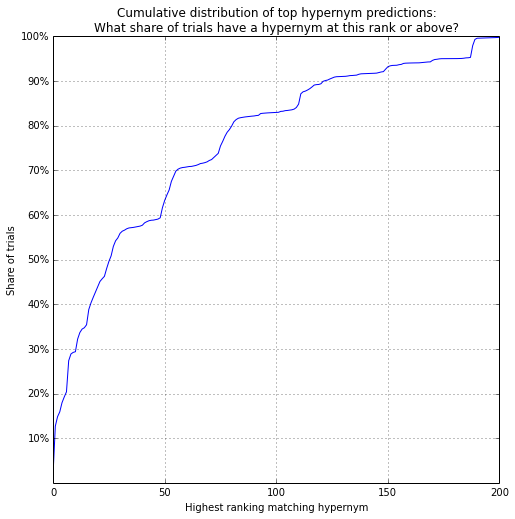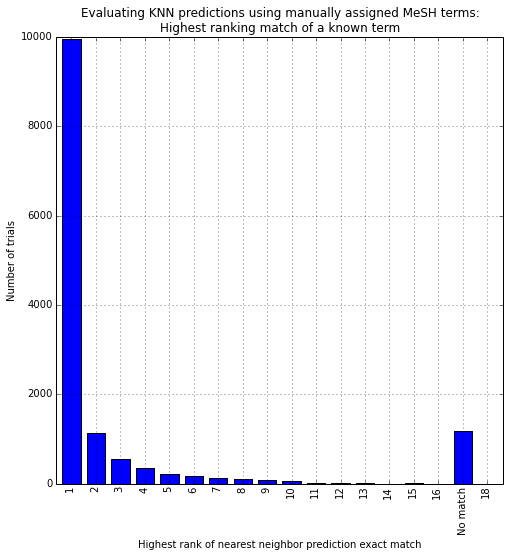In :
import codecs, string, random, math, cPickle as pickle, re, pandas as pd
from matplotlib.ticker import FuncFormatter
from collections import Counter
from IPython.display import HTML, Javascript, display

from __future__ import division

%matplotlib inline

In :
corrections = {"Sarcoma, Ewing's": 'Sarcoma, Ewing',
'Beta-Thalassemia': 'beta-Thalassemia',
'Von Willebrand Disease, Type 3': 'von Willebrand Disease, Type 3',
'Von Willebrand Disease, Type 2': 'von Willebrand Disease, Type 2',
'Von Willebrand Disease, Type 1': 'von Willebrand Disease, Type 1',
'Felty''s Syndrome': 'Felty Syndrome',
'Von Hippel-Lindau Disease': 'von Hippel-Lindau Disease',
'Retrognathism': 'Retrognathia',
'Regurgitation, Gastric': 'Laryngopharyngeal Reflux',
'Persistent Hyperinsulinemia Hypoglycemia of Infancy': 'Congenital Hyperinsulinism',
'Von Willebrand Diseases': 'von Willebrand Diseases',
'Pontine Glioma': 'Brain Stem Neoplasms',
'Mental Retardation': 'Intellectual Disability',
'Overdose': 'Drug Overdose',
'Beta-Mannosidosis': 'beta-Mannosidosis',
'Alpha 1-Antitrypsin Deficiency': 'alpha 1-Antitrypsin Deficiency',
'Intervertebral Disk Displacement': 'Intervertebral Disc Displacement',
'Alpha-Thalassemia': 'alpha-Thalassemia',
'Mycobacterium Infections, Atypical': 'Mycobacterium Infections, Nontuberculous',
'Legg-Perthes Disease': 'Legg-Calve-Perthes Disease',
'Intervertebral Disk Degeneration': 'Intervertebral Disc Degeneration',
'Alpha-Mannosidosis': 'alpha-Mannosidosis',
'Gestational Trophoblastic Disease': 'Gestational Trophoblastic Neoplasms'
}
cond = {}
cond_r = {}
row_id, trial_id, mesh_term = row.strip().split('|')
if mesh_term in corrections: mesh_term = corrections[mesh_term]
if mesh_term not in cond: cond[mesh_term] = []
cond[mesh_term].append(trial_id)
if trial_id not in cond_r: cond_r[trial_id] = []
cond_r[trial_id].append(mesh_term)

mesh_codes = {}
mesh_codes_r = {}
row_id, mesh_id, mesh_term = row.strip().split('|')
mesh_codes[mesh_id] = mesh_term
if mesh_term not in mesh_codes_r: mesh_codes_r[mesh_term] = []
mesh_codes_r[mesh_term].append(mesh_id)

# limiting to conditions that appear in ten or more trials
top_cond = {c for c in cond if len(cond[c]) >= 10}
trials = {t for c in top_cond for t in cond[c]}

# trial descriptions

# holdout sample text counters used in classification

In :
In :
print len(multi_preds)
print len(single_preds)
print len(knn_preds)
38075
38075
37527

Evaluating single-prediction model¶

What is the maximum overlap between the predicted term and the manually assigned terms?

Overlap is defined by the level of the least common hypernym in the MeSH hierarchy. A value of zero indicates that there is no overlap at all between the predicted and manually assigned terms.

In :
single_accuracy = {}
for trial_id in list(set(single_preds.keys()) & set(cond_r.keys()) & trials):
# initialize variables for this prediction
accuracy = 0
this_pred = single_preds[trial_id]
if this_pred in mesh_codes_r:
mesh_pred = mesh_codes_r[single_preds[trial_id]]

# loop through known MeSH terms to look for greatest overlap
for m in cond_r[trial_id]:
if m in mesh_codes_r:
for a in mesh_codes_r[m]:
len_a = len(a)
for i in range(len_a,accuracy,-4):
for pa in mesh_pred:
if pa[:i] == a[:i] and i > accuracy: accuracy = i

single_accuracy[trial_id] = int((accuracy + 1) / 4) if accuracy > 0 else 'No match'
In :
c = Counter(single_accuracy.values())
print sum(c.values())
ax = pd.DataFrame(c.values(), index=c.keys()).plot(kind='bar',
legend=False,
figsize=(8,8))
ax.set_xlabel("Hierarchy level")
ax.set_ylabel("Number of trials")
ax.set_title("Evaluating MaxEnt classification using manually assigned MeSH terms:\nMaximum depth of a matching term")
13965
Out:
<matplotlib.text.Text at 0x14c136090>Evaluating multiple prediction models¶

How well do the (accurate) level-2 hypernym predictions rank?

What is the highest (i.e., lowest number) rank of a manually assigned term's level-2 hypernym?

In :
mult_accuracy = {}
for trial_id in list(set(multi_preds.keys()) & set(cond_r.keys()) & trials):
# initialize variables for this prediction
top_rank = 10000
this_pred = {d: i for i, d in list(enumerate(sorted(multi_preds[trial_id].items(),
key=lambda x: x,
reverse=True)))
}

# loop through known MeSH terms to look for greatest overlap
for m in cond_r[trial_id]:
if m in mesh_codes_r:
for a in mesh_codes_r[m]:
if a[:7] in this_pred and this_pred[a[:7]] < top_rank: top_rank = this_pred[a[:7]]

if top_rank < 10000:
mult_accuracy[trial_id] = top_rank
In :
low_rank = max(mult_accuracy.values())
c = Counter(mult_accuracy.values())
print sum(c.values())

ticks = [k for k in range(low_rank) if k%10 == 0]

ax = pd.DataFrame([c[i] for i in range(low_rank+1)],
index=range(low_rank+1)).plot(kind='bar',
legend=False,
xticks=ticks,
figsize=(14,8))
ax.set_xticklabels(ticks)
ax.set_xlabel("Highest rank of matching hypernym")
ax.set_ylabel("Number of trials")
ax.set_title("Evaluating hypernym classifiers using manually assigned MeSH terms:\nHighest ranking hypernym of a known term")
13745
Out:
<matplotlib.text.Text at 0x196e25310>In :
total = sum(c.values())
ticks = [i/10 for i in range(1,11)]
ax = pd.DataFrame([sum([c[j] for j in c if j <= i]) / total for i in range(low_rank+1)],
index=range(low_rank+1)).plot(legend=False,
xlim=(0,200),
yticks=ticks,
figsize=(8,8))
ax.set_xlabel("Highest ranking matching hypernym")
ax.set_ylabel("Share of trials")
ax.set_yticklabels(['%d%%' % (r*100) for r in ticks])
ax.set_title("Cumulative distribution of top hypernym predictions:\nWhat share of trials have a hypernym at this rank or above?")
Out:
<matplotlib.text.Text at 0x15d975fd0>Evaluating KNN model¶

Does an exact match exist among the neighbors? At what rank?

If not, does a hypernym match exist? At what level and rank?

In :
knn_accuracy = {}
for trial_id in list(set(knn_preds.keys()) & set(cond_r.keys()) & trials):
# initialize variables for this prediction
accuracy = {'exact': 10000,
'hypernym': {}}
this_pred = dict([(k, sum([1 / (10 ** d) for d in v]))
for k, v in knn_preds[trial_id].items()
])
val_order = {j: i for i, j in enumerate(sorted(list(set(this_pred.values())), reverse=True))}

hyp_pred = {m: val_order[v]
for p, v in this_pred.items()
if p in mesh_codes_r
for m in mesh_codes_r[p]}

# loop through known MeSH terms to look for greatest overlap
for m in cond_r[trial_id]:
if m in this_pred:
this_rank = val_order[this_pred[m]]
if this_rank < accuracy['exact']:
accuracy['exact'] = this_rank
elif m in mesh_codes_r:
for a in mesh_codes_r[m]:
len_a = len(a)
for i in range(len_a,0,-4):
for pa in hyp_pred:
if pa[:i] == a[:i]:
if i not in accuracy['hypernym'] or hyp_pred[pa] < accuracy['hypernym'][i]:
accuracy['hypernym'][i] = hyp_pred[pa]

if accuracy['exact'] == 10000: accuracy['exact'] = None

knn_accuracy[trial_id] = accuracy
In :
c = Counter([knn_accuracy[t]['exact']+1
if knn_accuracy[t]['exact'] is not None
else 'No match'
for t in knn_accuracy.keys()])
print sum(c.values()), len(knn_accuracy)

ax = pd.DataFrame(c.values(), index=c.keys()).plot(kind='bar',
figsize=(8,8),
legend=False)

ax.set_xlabel("Highest rank of nearest neighbor prediction exact match")
ax.set_ylabel("Number of trials")
ax.set_title("Evaluating KNN predictions using manually assigned MeSH terms:\nHighest ranking match of a known term")
13959 13959
Out:
<matplotlib.text.Text at 0x1a596bfd0>Testing random instances¶

In :
holdout_sample = list(set(knn_preds.keys()) & set(cond_r.keys()) & trials)
rare_mesh = list(set(knn_preds.keys()) & set(cond_r.keys()) - trials)
unknown_mesh = list(set(knn_preds.keys()) - set(cond_r.keys()))
In :
def mesh_url(this_term, this_code):
this_term = this_term.replace(' ','+')
url = 'http://www.nlm.nih.gov/cgi/mesh/2015/MB_cgi?mode=&term=%s&field=entry#Tree%s' % (this_term, this_code)
return '<a target="_blank" href=%s>%s</a>' % (url, this_code)

def html_output(trial_id):

if trial_id in cond_r:
assigned = cond_r[trial_id]
assigned_codes = set()
for m in assigned:
if m in mesh_codes_r: assigned_codes |= set(mesh_codes_r[m])
assigned_hyp = set([c[:7] for c in assigned_codes if len(c) > 3])
else:
assigned = []

if trial_id in single_preds:
top_pred = single_preds[trial_id]
else:
top_pred = ''

if trial_id in knn_preds:
knn_pred = sorted([(k, sum([1 / (10 ** d) for d in v]))
for k, v in knn_preds[trial_id].items()
], key=lambda x: x, reverse=True)
knn_ranks = {}
rank = 1
for k,v in knn_pred:
if len(knn_ranks) == 0: cur_val = v
if v == cur_val:
knn_ranks[k] = rank
else:
rank += 1
cur_val = v
knn_ranks[k] = rank
rank_count = Counter(knn_ranks.values())
else:
knn_pred = []

if trial_id in multi_preds:
hyp_pred = sorted(multi_preds[trial_id].items(),
key=lambda x: x,
reverse=True)
top_hyps = [c for c, v in hyp_pred[:20]]
else:
top_hyps = []

def print_codes(cur_term, paint=False):
code_html = ''
if cur_term in mesh_codes_r:
cur_codes = mesh_codes_r[cur_term][:]
cur_codes.sort()
for code in cur_codes:
painting = ''
if paint and code[:7] in top_hyps:
painting = '&nbsp;<span style="background-color: #FF69BA; font-size: small;">(hypernym in top 20)</span>'
code_html += '<p style="padding-left: 30px; margin-top: 0px">%s%s</p>' % (mesh_url(cur_term,code),
painting)
return code_html

page = "<p><strong>Trial ID:</strong> %s</p>\n" % trial_id
page += "<h3>Brief description</h3><p>%s</p>\n" % trial_desc[trial_id]
page += "<h3>Detailed description</h3><p>%s</p>\n" % (trial_desc[trial_id]
if trial_desc[trial_id]
else "No detailed description.")
page += "<h2>Current MeSH assignment (if any)</h2>"
if assigned:
for t in assigned:
if t == top_pred:
font-weight: normal;
font-size: small;
font-style: normal">(same as top single prediction)</span>'''
if t in knn_ranks and knn_ranks[t] == 1:
font-weight: normal;
font-size: small;
font-style: normal">(top-ranked KNN prediction)</span>'''
elif t in knn_ranks:
font-weight: normal;
font-size: small;
font-style: normal">(KNN prediction)</span>'''
page += '<h5 style="margin-top: 8px;">%s%s</h5>' % (t,
page += print_codes(t, paint=True)
else:
page += '<p>No MeSH assignment.</p>'

page += '<h2>Top single prediction</h2>'
top_pred_style = ''
if top_pred in assigned:
top_pred_style = ' style="background-color: greenyellow;"'
page += '<h5 style="margin-top: 8px;"><span%s>%s</span></h5>' % (top_pred_style,
top_pred)
page += print_codes(top_pred)

page += '<h2>K Nearest Neighbor predictions</h2>'
for this_term, cur_val in knn_pred:
knn_style = ''
if this_term in assigned:
knn_style = ' style="background-color: %s;"' % ('lightsalmon' if knn_ranks[this_term] == 1 else '#FFD9CA')
knn_tie = ''
if rank_count[knn_ranks[this_term]] > 1:
knn_tie = ' (tie)'
page += '<h5 style="margin-top: 8px;">%d%s. <span%s>%s</span> </h5>' % (knn_ranks[this_term],
knn_tie,
knn_style,
this_term)
page += print_codes(this_term)

page += '<h2>Top 20 MeSH hypernym predictions</h2>'
hyp_rank = 1
for code in top_hyps:
hyp_style = ''
if code in assigned_hyp:
hyp_style = ' style="background-color: #FF69BA;"'
page += '<p style="margin-top: 0px">%d. <span style="font-weight: bold;">%s</span>' % (hyp_rank,
mesh_url(mesh_codes[code],code))
page += '&nbsp;&nbsp;<span%s>%s</span></p>' % (hyp_style,
mesh_codes[code])
hyp_rank += 1

return page
In :
display(HTML(html_output(random.choice(holdout_sample))))

Trial ID: NCT01404273

Brief description

This research study is being done to examine how meditation and the relaxation response (RR) may change brain activity in attention-deficit/hyperactivity disorder (ADHD).

Detailed description

No detailed description.

Current MeSH assignment (if any)

Hyperkinesis (KNN prediction)

C10.597.350.350 (hypernym in top 20)

C23.888.592.350.350 (hypernym in top 20)

F03.550.150.150

F03.550.150.150

K Nearest Neighbor predictions

F03.550.150.150

2. Hyperkinesis

C10.597.350.350

C23.888.592.350.350

C08.618.749

C23.550.773

C25.775.300

F03.900.300

C25.775

F03.900

Top 20 MeSH hypernym predictions

1. C23.300  Pathological Conditions, Anatomical

2. C23.888  Signs and Symptoms

3. C16.131  Congenital Abnormalities

4. C16.614  Infant, Newborn, Diseases

5. C01.539  Infection

6. C10.597  Neurologic Manifestations

7. C18.654  Nutrition Disorders

8. C23.550  Pathologic Processes

9. C05.660  Musculoskeletal Abnormalities

10. C14.240  Cardiovascular Abnormalities

11. G07.100  Body Constitution

12. C10.228  Central Nervous System Diseases

13. C09.218  Ear Diseases

14. E01.370  Diagnostic Techniques and Procedures

15. C13.703  Pregnancy Complications

16. C10.500  Nervous System Malformations

17. C01.252  Bacterial Infections

18. F01.700  Neurobehavioral Manifestations

19. C14.907  Vascular Diseases

20. C10.900  Trauma, Nervous System

In [ ]: